## Recent Posts

▸ Linear regression and get to see it work on data.

I have recently completed the Machine Learning course from Coursera by Andrew NG.

While doing the course we have to go through various quiz and assignments.

Here, I am sharing my solutions for the weekly assignments throughout the course.

These solutions are for reference only.

> It is recommended that you should solve the assignments by yourself honestly then only it makes sense to complete the course.
> But, In case you stuck in between, feel free to refer to the solutions provided by me.

#### NOTE:

Don't just copy paste the code for the sake of completion.
Even if you copy the code, make sure you understand the code first.

Since there is NO assignment in week-1, Let's start with the week-2 assignment....

In this exercise, you will implement linear regression and get to see it work on data. Before starting on this programming exercise, we strongly recommend watching the video lectures and completing the review questions for the associated topics.

It consist of the following files:
• ex1.m - Octave/MATLAB script that steps you through the exercise
• ex1 multi.m - Octave/MATLAB script for the later parts of the exercise
• ex1data1.txt - Dataset for linear regression with one variable
• ex1data2.txt - Dataset for linear regression with multiple variables
• submit.m - Submission script that sends your solutions to our servers
• Simple example function in Octave/MATLAB
• Function to display the dataset
• Function to compute the cost of linear regression
• Function to run gradient descent
• Cost function for multiple variables
• Gradient descent for multiple variables
• Function to normalize features
• Function to compute the normal equations
• YouTube videos featuring Free IOT/ML tutorials
* indicates files you will need to complete
# indicates optional exercises

### warmUpExercise.m :

```function A = warmUpExercise()
%WARMUPEXERCISE Example function in octave
%   A = WARMUPEXERCISE() is an example function that returns the 5x5 identity matrix

A = [];
% ============= YOUR CODE HERE ==============
% Instructions: Return the 5x5 identity matrix
%               In octave, we return values by defining which variables
%               represent the return values (at the top of the file)
%               and then set them accordingly.

A = eye(5);  %It's a built-in function to create identity matrix

% ===========================================
end```

### plotData.m :

```function plotData(x, y)
%PLOTDATA Plots the data points x and y into a new figure
%   PLOTDATA(x,y) plots the data points and gives the figure axes labels of
%   population and profit.

figure; % open a new figure window

% ====================== YOUR CODE HERE ======================
% Instructions: Plot the training data into a figure using the
%               "figure" and "plot" commands. Set the axes labels using
%               the "xlabel" and "ylabel" commands. Assume the
%               population and revenue data have been passed in
%               as the x and y arguments of this function.
%
% Hint: You can use the 'rx' option with plot to have the markers
%       appear as red crosses. Furthermore, you can make the
%       markers larger by using plot(..., 'rx', 'MarkerSize', 10);

plot(x, y, 'rx', 'MarkerSize', 10); % Plot the data
ylabel('Profit in \$10,000s'); % Set the y-axis label
xlabel('Population of City in 10,000s'); % Set the x-axis label

% ============================================================
end```

### computeCost.m :

```function J = computeCost(X, y, theta)
%COMPUTECOST Compute cost for linear regression
%   J = COMPUTECOST(X, y, theta) computes the cost of using theta as the
%   parameter for linear regression to fit the data points in X and y

% Initialize some useful values
m = length(y); % number of training examples

% You need to return the following variables correctly
J = 0;

% ====================== YOUR CODE HERE ======================
% Instructions: Compute the cost of a particular choice of theta
%               You should set J to the cost.

%%%%%%%%%%%%% CORRECT %%%%%%%%%
% h = X*theta;
% temp = 0;
% for i=1:m
%   temp = temp + (h(i) - y(i))^2;
% end
% J = (1/(2*m)) * temp;
%%%%%%%%%%%%%%%%%%%%%%%%%%%%%%%

%%%%%%%%%%%%% CORRECT: Vectorized Implementation %%%%%%%%%
J = (1/(2*m))*sum(((X*theta)-y).^2);
%%%%%%%%%%%%%%%%%%%%%%%%%%%%%%%%%%%%%%%%%%%%%%%%%%%%%%%%%%

% =========================================================================
end```

```function [theta, J_history] = gradientDescent(X, y, theta, alpha, num_iters)
%GRADIENTDESCENT Performs gradient descent to learn theta
%   theta = GRADIENTDESCENT(X, y, theta, alpha, num_iters) updates theta by
%   taking num_iters gradient steps with learning rate alpha

% Initialize some useful values
m = length(y); % number of training examples
J_history = zeros(num_iters, 1);

for iter = 1:num_iters

% ====================== YOUR CODE HERE ======================
% Instructions: Perform a single gradient step on the parameter vector
%               theta.
%
% Hint: While debugging, it can be useful to print out the values
%       of the cost function (computeCost) and gradient here.
%

%%%%%%%%% CORRECT %%%%%%%
%error = (X * theta) - y;
%temp0 = theta(1) - ((alpha/m) * sum(error .* X(:,1)));
%temp1 = theta(2) - ((alpha/m) * sum(error .* X(:,2)));
%theta = [temp0; temp1];
%%%%%%%%%%%%%%%%%%%%%%%%%

%%%%%%%%% CORRECT %%%%%%%
%error = (X * theta) - y;
%temp0 = theta(1) - ((alpha/m) * X(:,1)'*error);
%temp1 = theta(2) - ((alpha/m) * X(:,2)'*error);
%theta = [temp0; temp1];
%%%%%%%%%%%%%%%%%%%%%%%%%

%%%%%%%%% CORRECT %%%%%%%
error = (X * theta) - y;
theta = theta - ((alpha/m) * X'*error);
%%%%%%%%%%%%%%%%%%%%%%%%%

% ============================================================

% Save the cost J in every iteration
J_history(iter) = computeCost(X, y, theta);

end
end```

### computeCostMulti.m :

```function J = computeCostMulti(X, y, theta)
%COMPUTECOSTMULTI Compute cost for linear regression with multiple variables
%   J = COMPUTECOSTMULTI(X, y, theta) computes the cost of using theta as the
%   parameter for linear regression to fit the data points in X and y

% Initialize some useful values
m = length(y); % number of training examples

% You need to return the following variables correctly
J = 0;

% ====================== YOUR CODE HERE ======================
% Instructions: Compute the cost of a particular choice of theta
%               You should set J to the cost.

J = (1/(2*m))*(sum(((X*theta)-y).^2));

% =========================================================================

end```

```function [theta, J_history] = gradientDescentMulti(X, y, theta, alpha, num_iters)
%GRADIENTDESCENTMULTI Performs gradient descent to learn theta
%   theta = GRADIENTDESCENTMULTI(x, y, theta, alpha, num_iters) updates theta by
%   taking num_iters gradient steps with learning rate alpha

% Initialize some useful values
m = length(y); % number of training examples
J_history = zeros(num_iters, 1);

for iter = 1:num_iters

% ====================== YOUR CODE HERE ======================
% Instructions: Perform a single gradient step on the parameter vector
%               theta.
%
% Hint: While debugging, it can be useful to print out the values
%       of the cost function (computeCostMulti) and gradient here.
%

%%%%%%%% CORRECT %%%%%%%%%%
error = (X * theta) - y;
theta = theta - ((alpha/m) * X'*error);
%%%%%%%%%%%%%%%%%%%%%%%%%%%

% ============================================================

% Save the cost J in every iteration
J_history(iter) = computeCostMulti(X, y, theta);

end
end```

### featureNormalize.m :

```function [X_norm, mu, sigma] = featureNormalize(X)
%FEATURENORMALIZE Normalizes the features in X
%   FEATURENORMALIZE(X) returns a normalized version of X where
%   the mean value of each feature is 0 and the standard deviation
%   is 1. This is often a good preprocessing step to do when
%   working with learning algorithms.

% You need to set these values correctly
X_norm = X;
mu = zeros(1, size(X, 2));
sigma = zeros(1, size(X, 2));

% ====================== YOUR CODE HERE ======================
% Instructions: First, for each feature dimension, compute the mean
%               of the feature and subtract it from the dataset,
%               storing the mean value in mu. Next, compute the
%               standard deviation of each feature and divide
%               each feature by it's standard deviation, storing
%               the standard deviation in sigma.
%
%               Note that X is a matrix where each column is a
%               feature and each row is an example. You need
%               to perform the normalization separately for
%               each feature.
%
% Hint: You might find the 'mean' and 'std' functions useful.
%

mu = mean(X);
sigma = std(X);
X_norm = (X - mu)./sigma;

% ============================================================
end```

### normalEqn.m :

```function [theta] = normalEqn(X, y)
%NORMALEQN Computes the closed-form solution to linear regression
%   NORMALEQN(X,y) computes the closed-form solution to linear
%   regression using the normal equations.

theta = zeros(size(X, 2), 1);

% ====================== YOUR CODE HERE ======================
% Instructions: Complete the code to compute the closed form solution
%               to linear regression and put the result in theta.
%

% ---------------------- Sample Solution ----------------------

theta = pinv(X'*X)*X'*y;

% -------------------------------------------------------------
% ============================================================
end```

I tried to provide optimized solutions like vectorized implementation for each assignment. If you think that more optimization can be done, then put suggest the corrections / improvements.

--------------------------------------------------------------------------------
Click here to see solutions for all Machine Learning Coursera Assignments.
&
Click here to see more codes for Raspberry Pi 3 and similar Family.
&
Click here to see more codes for NodeMCU ESP8266 and similar Family.
&
Click here to see more codes for Arduino Mega (ATMega 2560) and similar Family.

Feel free to ask doubts in the comment section. I will try my best to solve it.
If you find this helpful by any mean like, comment and share the post.
This is the simplest way to encourage me to keep doing such work.

Thanks and Regards,
-Akshay P. Daga

1.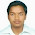Have you got prediction values as expected?

1.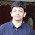Yes. We got prediction values as expected.

2.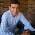Thanks for your comments. I still have some problems with the solutions, could you help me. In this case is with line 17, J History....

Week 2
function [theta, J_history] = gradientDescent(X, y, theta, alpha, num_iters)
%GRADIENTDESCENT Performs gradient descent to learn theta
% theta = GRADIENTDESCENT(X, y, theta, alpha, num_iters) updates theta by
% taking num_iters gradient steps with learning rate alpha

% Initialize some useful values

X = data(:,1)
y = data(:,2)
m = length(y)
x = [ones(m, 1), data(:,1)]
theta = zeros(2, 1)
iterations = 1500
alpha = 0.01
J = (1 / (2* m) ) * sum(((x* theta)-y).^2)
J_history = zeros(num_iters, 1)

for iter = 1:num_iters

% ====================== YOUR CODE HERE ======================
% Instructions: Perform a single gradient step on the parameter vector
% theta.
%
% Hint: While debugging, it can be useful to print out the values
% of the cost function (computeCost) and gradient here.
%

%error = (X * theta) - y;
%temp0 = theta(1) - ((alpha/m) * sum(error .* X(:,1)));
%temp1 = theta(2) - ((alpha/m) * sum(error .* X(:,2)));
%theta = [temp0; temp1];

% ============================================================

% Save the cost J in every iteration
J_history(iter) = computeCost(X, y, theta);

end

end

3.error: 'y' undefined near line 7 column 12
error: called from
gradientDescent at line 7 column 3
>> computeCost()
error: 'y' undefined near line 7 column 12
error: called from
computeCost at line 7 column 3

How to correct this?

1.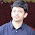I tried to re-ran the code and everything worked perfectly fine with me.
Please check you code.
In the code, you can variable "y" is defined in parameter list itself.
So, logically you should not get that error.
There must something else you might be missing outside these functions.

4.computeCost

error: 'y' undefined near line 8 column 12
error: called from
computeCost at line 8 column 3

error: 'y' undefined near line 7 column 12
error: called from
gradientDescent at line 7 column 3

How to correct this?

1.I tried to re-ran the code and everything worked perfectly fine with me.
Please check you code.
In the code, you can variable "y" is defined in parameter list itself.
So, logically you should not get that error.
There must something else you might be missing outside these functions.

If you got the solution please confirm here. It will be helpful for others.

2.Hi,
Receiving similar error. Found a solution?

3.Hello,
Got a similar error!
found the solution?

4.Hello,
Got a similar error!
found the solution?

5.Hi Sasank,
because small y already is used as a input argument for the mentioned functions. So, you can't get the error like y is undefined.
Are you sure you haven't made any mistake like small y and Capital Y ?
Please check it and try again.

6.error: 'X' undefined near line 9 column 10
error: called from
featureNormalize at line 9 column 8

5.I was stuck for two months in Week 2 Assignment of Machine Learning . Thanx for your guidance due to which I can now understand coding in a better way and finally I have passed 2nd Week Assignment.

1.Glad to know that my work helped you in understanding the topic / coding.
You can also checkout free IOT tutorials with source codes and demo here: https://www.apdaga.com/search/label/IoT%20%28Internet%20of%20Things%29
Thanks.

6.I tried to reran the code. But i am getting error this:
error: 'num_iters' undefined near line 17 column 19
error: called from
gradientDescent at line 17 column 11
how to correct this??

7.i am also submitting these assignments . i have also done the same . But i dont know where to load data .thus my score is 0. how can i improve? please suggest me.

1.Refer the forum within the course in Coursera.

They have explained the step to submit the assignments in datails.

8.Hello ,

In the gradient descent.m file : theta = theta - ((alpha/m) * X'*error);
I m confused, why do we take the transpose of X (X'*error) instead
of X ?

B

1.Hi Bruno,
I got your confusion, Here X (capital X) represent all the training data together, each row as one training sample, each column as a feature. We want to multiply each training data with corresponding error. To make it happen you have to transpose of X (capital X).

if you take x (small x) as single training sample then you don't have to worry about transpose and all.
Simply (x * error) will work.

Try to do it manually on a notebook. You will understand it.

2.Hi Akshay

Thank you for the quick reply & help ...It s totally clear now, make sense !!!

Have a great day
Bruno

9.Good day,please am kind of stuck with week2 programming assignment,and this is under computecost.m
I already imported the data and plotted the scatter plot.Do I also after to import the data in computecost.m working area,and and when I just in inputted the code J = (1/(2*m))*(sum(((X*theta)-y).^2)); I got an error message.please how do I fix this.
Thanks

1.What error you got?

10.plotData

error: 'X' undefined near line 20 column 6
error: called from
plotData at at line 20 column 1

What is the solution to this?

1.Hi Amit, As I checked I have used small x as an input argument for plotData function.
and in your error there is capital X.
Are you sure you haven't made mistake in small and capital X?
Please check and try once again.

2.i can see you have used a X there not x,still showing the error saying not enough input arguments

11.Hey Akshay, The error 'y' undefined problem do exist, but it is not othe problem only for the code you gave,any solution the internet gives that error.Even running through gui or through command, it says undefined.There is no clear solution for this on the net, I tried adding path too as it was said in the net.Couldnt solve the issue.I have octave 5.1.0

1.I found the solition for those who were getting u defi ed error.
if you are using octave
then
the file shouldnot first start with function, octave takes it as a function, not as a script.

solution
add anything to first line
example
add 1; first line and then start function.

If you wanna test your function when you run, first initialize the variables to matrices and respective values.
then pass these as parameters to the function.

2.Thanks Chethan,
It will be a great help for others as well.

3.I didn't understand.can u explain clearly

4.include two lines of code

x=[];
y=[];

This should work

12.Hi Akshay,
I am getting error like this
m=lenght(y); %number of training example

Can you help me

Thanks

13.This comment has been removed by a blog administrator.

14.Hello, within gradientDescent you use the following code

error = (X * theta) - y;
theta = theta - ((alpha/m) * X'*error)

What is the significance of 'error' in this? Within Ng's lectures I can't remember him making reference to 'error'

1.Error is similar to that of "cost" (J)

15.!! Submission failed: 'data' undefined near line 19 column 18

LineNumber: 19

This is my problem how to correct it

1.Hi, I think you are doing this assignment in Octave and that's why you are facing this issue.

Chethan Bhandarkar has provided solution for it. Please check out the comment by Chethan Bhandarkar: https://www.apdaga.com/2018/06/coursera-machine-learning-week-2.html?showComment=1563986935868#c4682866656714070064

Thanks

16.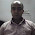Code that is given is not running as always give error 'y' undefined near line 7 column 12 for every code.

1.Hi, I think you are doing this assignment in Octave and that's why you are facing this issue.

Chethan Bhandarkar has provided solution for it. Please check out the comment by Chethan Bhandarkar: https://www.apdaga.com/2018/06/coursera-machine-learning-week-2.html?showComment=1563986935868#c4682866656714070064

Thanks

17.18.